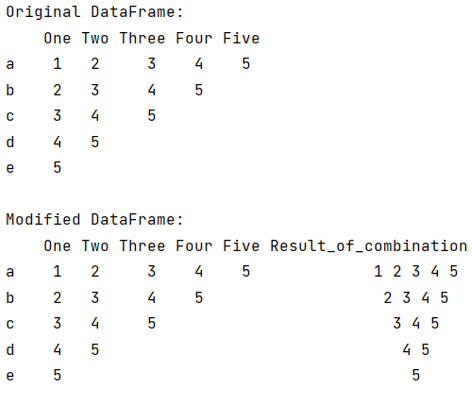# Merge multiple column values into one column in Python pandas

Given a Pandas DataFrame, we need to combine all the values of a column and append them into another single column.
Submitted by Pranit Sharma, on July 26, 2022

Pandas is a special tool that allows us to perform complex manipulations of data effectively and efficiently. Inside pandas, we mostly deal with a dataset in the form of DataFrame. DataFrames are 2-dimensional data structures in pandas. DataFrames consist of rows, columns, and data.

To combine the values of all the column and append them into a single column, we will use apply() method inside which we will write our expression to do the same. Whenever we want to perform some operation on the entire DataFrame, we use apply() method.

The apply() method clearly passes the columns of each group in the form of a DataFrame inside the function which is described in apply() method. Here, we will define a lambda function inside the apply() method.

Let us understand with the help of an example,

## Python code to merge multiple column values into one column

```# Importing pandas package
import pandas as pd

# Creating a Dictionary
d = {
'One':[1,2,3,4,5],
'Two':[2,3,4,5,''],
'Three':[3,4,5,'',''],
'Four':[4,5,'','',''],
'Five':[5,'','','','']
}

# Creating a DataFrame
df = pd.DataFrame(d,index=['a','b','c','d','e'])

# Display original DataFrame
print("Original DataFrame:\n",df,"\n")

# Using apply method to combine values
df['Result_of_combination'] = df[df.columns[0:]].apply(lambda x: ' '.join(x.dropna().astype(str)),axis=1)

# Display modified DataFrame
print("Modified DataFrame:\n",df)
```

Output:Languages: » C » C++ » C++ STL » Java » Data Structure » C#.Net » Android » Kotlin » SQL
Web Technologies: » PHP » Python » JavaScript » CSS » Ajax » Node.js » Web programming/HTML
Solved programs: » C » C++ » DS » Java » C#
Aptitude que. & ans.: » C » C++ » Java » DBMS
Interview que. & ans.: » C » Embedded C » Java » SEO » HR
CS Subjects: » CS Basics » O.S. » Networks » DBMS » Embedded Systems » Cloud Computing
» Machine learning » CS Organizations » Linux » DOS
More: » Articles » Puzzles » News/Updates